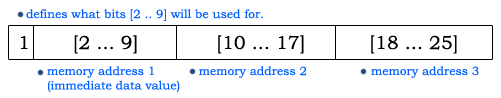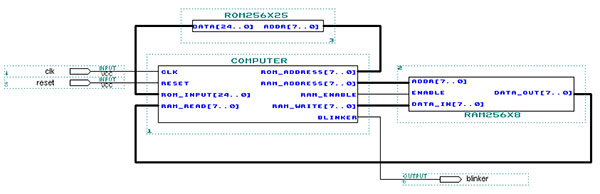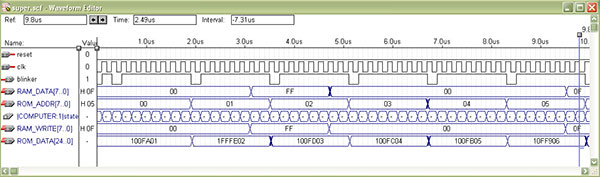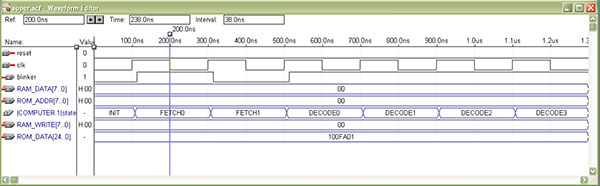E25 Computer Architecture: Lab 5
One Instruction Set Computer (OISC)
in partnership with mustafa paksoy
05.16.2005

1. Abstract.
In this lab, we designed and implemented a One Instruction Set Computer. The instruction we used was "SUBLEQ A B C" which means "subtract the value in M(A) from M(B) and store it in M(B), if the result is not positive, go to instruction C". In order to be able to store values, we also added a flag that allows us to save immediate values to a given location in memory. In order to implement our computer, we first developed a state machine, then we coded it up in VHDL and tested it on an Altera board. In addition we implemented an assembler and a virtual OISC machine.

2. OISC.
Our computer was very similar to the one we created in lab 3. The main differences were that, this implementation didn't have most of the registers the previous computer had. The main idea behind the OISC is to create a very simple computer that is fast. The OISC goes through the same routine for every instruction and the idea is to eliminate the decode stages found in other computers.

OISC used a 256 x 8 RAM which was implemented using the lpm_ram_dq() superfunction in VHDL. The RAM we used was asynchronous and allowed us to read only one instruction every cycle. We tried to implement a synchronous dual ram, but we weren't satisfied with the results because it took a lot of time to get fetch the data (it would take roughly 4 cycles to receive any data. Perhaps, running the RAM and the computer on two different clocks could speed up the process, but we were satisfied with the performance of our asynchronous RAM).

For the 256 x 25 ROM, we used the lpm_rom() superfunction. Figure 1 shows the parts of our 25-bit instruction.Figure 1. Parts of the 25-bit instruction.

Once the design of each part (main computer, RAM, ROM) we put everything together using the graphical tools in MAXII+. We put a blinker to signal fetches from the ROM (the project wouldn't compile without an output)Figure 2. Parts of the computer includes the main unit, RAM and ROM. click on image to see a larger version.

We used the waveform editor to test our computer. Figures 3 and 4 show some details from the testing. Please click on images for detailed versions.Figure 3. Execution of several sample instructions.Figure 4. Details of the execution states.

3. OISC compiler

For the second part of our final lab we wrote a compiler that uses our One Instruction Set Computer (OISC) chip. We came up with a simple programming language that permits variable definitions, since that permits variable definitions, and performs operations using addresses reserved for those variables. This provided a user-friendly interface to our CPU which only accepts memory addresses as operands.

3.1 Basic Characteristics

1) Compiler gives the address of the next line as the branch destination for non-branching instructions.

Example:
00000001 : 11111111111111110 00000010 ;
00000010 : 1000000001111110100000011 ;

2) The load immediate instruction is only used in the beginning of the ROM for RAM initialization. All algorithms only use the single “subtract direct and branch if <= 0” instruction, so our CPU essentially acts as an OISC. The second instruction only exists because we had no other way of initially loading operands to RAM.

3) Compiler is run from command line using:

java compileOISC <source file> [target file]

If no target file is specified default is “compiled.mif”.

## 3.2 Implemented Operations

SUB a b c: m(c) = m(b) - m(a)
ADD a b c: m(c) = m(a) + m(b)
DIV a b c: m(c) = m(a) / m(b)
MUL a b c: m(c) = m(a) * m(b)
IFGT a b c: IF a>b THEN JMP c
IFLE a b c: IF a<=b THEN JMP c
DEF x constant: define variable 'x' to be constant
MOV a b: move m(a) to m(b)

Except for the DEF, all operators in the compiler only accept direct memory addresses as operands. DEF accepts immediate values and enables variable definitions and this is the only operator that uses the load immediate instruction. All other operators are implemented only using the default instruction which only accepts memory addresses as operands. In our programming language, variable definitions allow user to ignore inconvenient memory addresses and when given as operands to operators, the addresses of variables are passed on to functions.

In the following description, when branch jump address (third operand of the subleq instruction) is not specified, jump to next line in ROM is assumed. When jump address is specified as +/-n, jump address is equal to current line in ROM +/- n. By this convention, by default jump address is +1.

ZR denotes the reserved register for the number zero, NR denotes the reserved register for the number negative one, TA TB TC are temporary storage registers A, B and C.

 ADD a b c SUB a b c MOV a b subleq a ZR subleq b ZR     // ZR = - a - b subleq c c      // c = 0 subleq ZR c     // c = a +b sublez ZR ZR    // ZR = 0 subleq TA TA            //TA = 0 subleq TB TB            //TB = 0 subleq a TA subleq b TB subleq TB TA            //TA = b - a subleq c c subleq TB TB subleq TA TB            //TB = a - b sublew TB c             //c = b – a subleq b b subleq a ZR subleq ZR b subleq ZR ZR JMPI a IFLE a b c       (if less than or equal to) IFGT a b c              (if greater than) subleq ZR a subleq TA TA subleq TA ZR subleq ZR ZR TA subleq TA TA subleq a TA             //TA = -a subleq TB TB subleq TA TB            //TB = a subleq b TB c           // TB = a – b, if (a-b)<=0 JMP c subleq TA TA subleq a TA             //TA = -a subleq TB TB subleq b TB             //TB = -b subleq TB TA            //TA = b – a subleq NR TB c   //TB = b – a + 1, if (b-a+1)<=0 JMP c

 DIV a b c MUL a b c subleq TA subleq TB subleq TC subleq b TA subleq TA TB            //TB = b subleq TA TA subleq a TA subleq TA TC            //TC = a subleq c c subleq NR c             //c++ subleq TC TB +2 //b -= a, if b<=0 JMP 2 subleq ZR ZR -2 //loop back to c++ subleq TA subleq TB subleq TC subleq b TA subleq TA TB            //TB = b subleq NR c             //c = 1 subleq TA TA subleq a TA             //TA = -a subleq c c //sub (-a) from dest and b--, when b<=0 esc subleq TA c subleq TC TB +2 subleq ZR ZR -2 //loop back

## 3.3 Compiler Behavior

Here is a demonstration of ROM state generation for some compiler capabilities.

3.3.1 RAM Initialization

The compiler reserves some of the highest RAM addresses as temporary registers and for storage of useful values such as zero (0x00) and negative one (0xFF). So regardless of contents of the source file the first few lines of ROM uses the load immediate instruction to initialize these reserved addresses.

Source: empty

Relevant ROM lines:

00000000 : 1 00000000 11111010 00000001 ;
00000001 : 1 11111111 11111110 00000010 ;
00000010 : 1 00000000 11111101 00000011 ;
00000011 : 1 00000000 11111100 00000100 ;
00000100 : 1 00000000 11111011 00000101 ;
00000101 : 1 00000000 11111111 00000110 ;

Immediate values are underlined, destination addresses are highlighted.

3.3.2 Variable Define

Variables can only defined in the beginning of file. The compiler reserves memory to them and initializes that memory location with the value they are assigned to.

Source:

DEF a 2
DEF b 2

Relevant ROM lines:

00000110 : 1 00000010 11111001 00000111 ;
00000111 : 1 00000011 11111000 00001000 ;

Memory location 11111001 is allocated to variable “a” and location is initialized with value 00000010(number 2).
Memory location 11111000 is allocated to variable “b” and location is initialized with value 00000011(number 3).

3.3.3 Jump Instruction

An instruction can be used to force jump to a location in ROM. This capability demonstrates how reserved registers are used as dummy operands.

Source:

JMP #01111110

Relevant ROM line:

00000110 : 0 1111101011111010 01111110 ;

The compiler uses the reserved register that contains zero as both operands (address underlined) and places the jump location in the branching address (highlighted). This will result in an unconditional jump.

4. virtual OISC machine.

We also wrote a program that can read .mif files and emulates the behavior of the OISC chip. This provided us with a convenient platform for testing algorithms we wrote in the compiler. The program can be run from command line using:

java virtualOISC

For more information on the programming and the usage of the Virtual OISC machine go here.

## 3.1 Demonstration

We loaded the compiled ROM state into the virtual machine, and initialized the RAM. We then compared the contents of RAM before and after running the program in ROM and determined whether memory had been properly modified. Here are the results of testing some of the operations available in our programming language.

### Subtract

Source code:

DEF a 90
DEF b -23
DEF c -51
SUB a b c # c = b - a = -113

(text after # is commented out)

As can be seen on highlighted lines, variable ‘c’ is initially equal to -51 (value assigned to be able to determine what memory address contains c) then it is overridden with -113, which is the correct result of operation -23 – 90. Some other addresses change, these are temporary registers which store intermediate values. [screenshot]

Source code:

DEF a 90
DEF b -23
DEF c -51
ADD a b c # c = b + a = 67

Once again variable ‘c’ is overridden with the appropriate value -23 + 90 = 67. [screenshot]

### Multiplication

Source code:

DEF a 30
DEF b 4
DEF c -51
MUL a b c # c = b * a = 120

Once again the right value is overridden to variable ‘c’ 30*4=120. [screenshot]

### Division

DEF a 4
DEF b 36
DEF c -51
DIV a b c # c = b / a = 9